Searching and Inserting with Red-Black Trees

Remember, given that red-black trees are binary trees in symmetric order, the process used to search a red-black tree for a given key is exactly the same as that used in a binary search tree. We can thus transfer the get(Key key) method from our binary search tree class to our red-black tree class, verbatim:

public Value get(Key key) {

Node n = root;

while (n != null) {
int cmp = key.compareTo(n.key);

if (cmp < 0)        // key < n.key
n = n.left;

else if (cmp > 0)   // key > n.key
n = n.right;

else                // key == n.key   (found it!)
return n.val;
}

}

Where things get more interesting is when it comes to insertion.

We start the insertion process by navigating down the tree to the node where the key must first be inserted. That much, at least, is straight-forward and can be modeled after the put() method used in our binary search tree class.

However, unlike in a binary search tree, we can't always just create a new node and append it where we would like it to go.

Granted, sometimes this is possible -- consider for example adding $G$ to the below tree.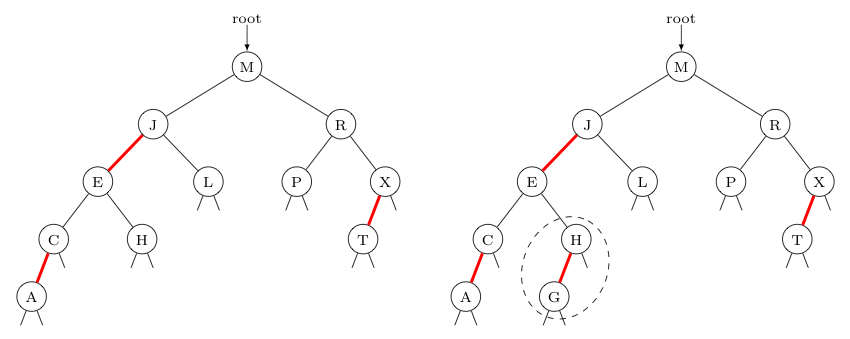In the case above, we only need to worry about the breaking of the perfect black balance of the tree when we add node $G$ under node $H$ -- a concern easily addressed by making the corresponding link red. Note, in terms of the underlying 2-3 tree, we just turned the 2-node $H$ into a 3-node $GH$.

Indeed, with the exception of adding the root node to a null tree, we can never add a node using a black edge -- as this would break the perfect black balance of the tree.

As such, we plan to make every new edge red. However, this can cause problems itself...

Consider the four cases below, where we insert a new key in the same place where a binary search tree would place it, but using a red edge -- and the problems these actions create. Images of the tree before and after the node was added are also shown for clarity.

Key To
Insert
Location Using
Binary Search Method
Problem Created
$Q$
right child of $P$
Adding this as right child creates a right-leaning edge in a left-leaning red-black tree.Key To
Insert
Location Using
Binary Search Method
Problem Created
$D$
right child of $C$
Not only did we add right-leaning red edge (which is its own problem), but also -- upon seeing a group of 3 keys connected by two adjacent red edges -- we see that a temporary 4-node has been created. To convince yourself this represents a 4-node, count the black links at the bottom of the $ACD$ group. This 4-node will need to be eliminated.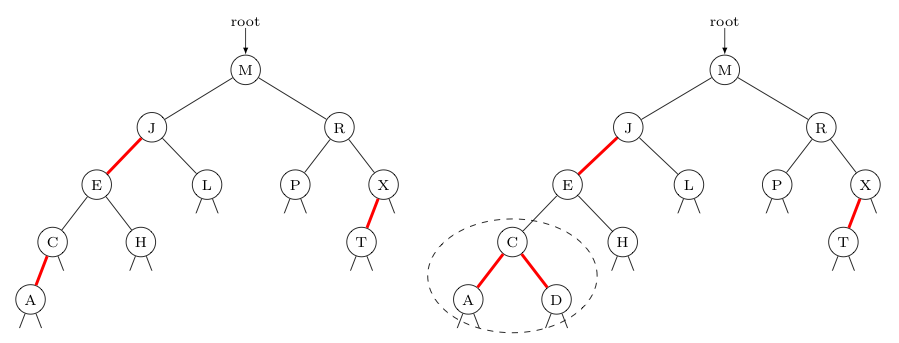Key To
Insert
Location Using
Binary Search Method
Problem Created
$S$
left child of $T$
In this case, we have no right-leaning red edges, but we still create a group of 3 keys connected by two adjacent red edges. This again creates a 4-node, which will need to be eliminated.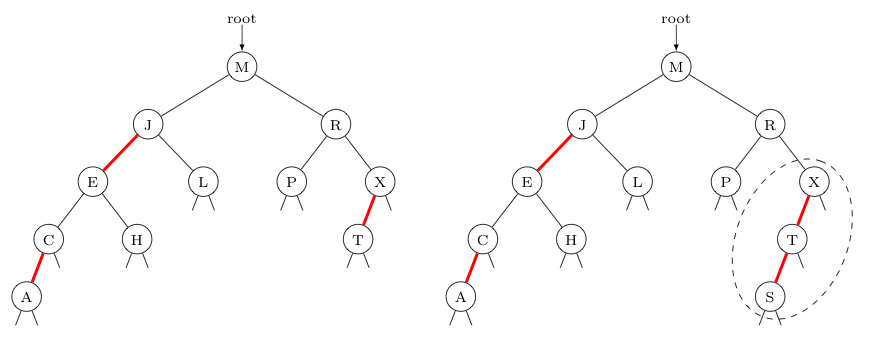Key To
Insert
Location Using
Binary Search Method
Problem Created
$U$
right child of $T$
Here, we again have the trouble-some right-leaning red edge. We also again have a 4-node created by a group of 3 keys connected by two adjacent red edges, that will need to be eliminated.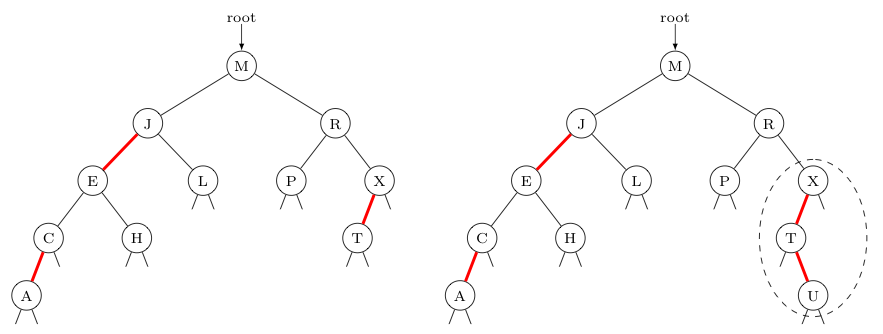Indeed, if the binary-search-tree insertion of a new node using a red edge into a red-black tree is going to cause a problem it will be similar to one of the 4 different cases shown above. As such, we need to figure out how to fix the following four "problem pieces":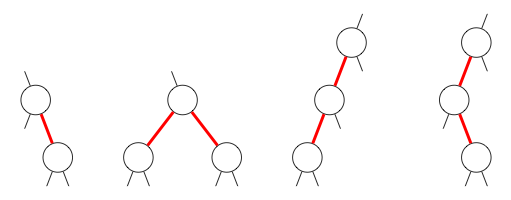Importantly, the last three of the above problem pieces are really just different realizations of 4-nodes.

Recall the process by which 4-nodes are eliminated in a 2-3 tree. We split the 4-node in two and pass the middle key to the parent of the former 4-node. This, of course may cause another 4-node higher up the tree, which in turn must be fixed. Indeed, such problems can track all the way to the root node in some cases.

It should be no surprise then, the strategy we will employ to fix the above problem pieces will often result in just moving the problem up a level, so that we can address it later. As fixing the problem may be "delayed" in this way multiple times, there is a chance that rather than seeing the null links shown with the pieces above, there may be large subtrees in their place. As such, any "fix" we use needs to work in these cases as well.

With this in mind, let us consider how to fix each of these 4 problem pieces (with subtrees) in turn. To make our examples concrete, let us assume (arbitrarily) the keys involved are $A$, $E$, and $S$.

Fixing "Lonely" Right-Leaning Red Edges"lonely" right-leaning red edgeThree of the four problem pieces above involve right-leaning red edges, but only one of these involves a right-leaning red edge not adjacent to some other red edge. This "lonely" right-leaning red edge can be converted to left-leaning red edges through the action of left rotation, described by the code and images below.

To see why this is called a rotation, imagine you disconnected $E$ from its parent and then rotated things rigidly in a counter-clockwise direction (as suggested by the curved blue arrow) so that $S$ could now become the child of $E$'s former parent. Note, as $E$ then becomes $S$'s left child, we must let $E$ "adopt" $S$'s prior left child as its new right child.

Before Left RotationAfter Left Rotation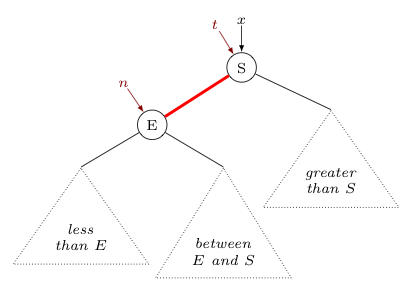private Node rotateLeft(Node n) {

assert isRed(n.right);  // only use rotateLeft when n's right link is red!

Node t = n.right;
n.right = t.left;
t.left = n;
t.color = n.color;
n.color = RED;
return t;
}

...

// then supposing the parent reference to $E$ is x,
// invoke with the following to update x

x = rotateLeft(x);

Fixing Adjacent Red Edges on the Same Leveladjacent red edges on the same levelConsider the case when we have two adjacent red edges on the same level in the tree, with a black edge connecting the node between them with its parent, as shown on the right.

As has been mentioned before, this is actually a 4-node in the associated 2-3 tree.

Following the strategy for insertion in a 2-3 tree, we split the 4-node and pass the middle node up to the parent of the former 4-node. This is easily accomplished by changing the colors of all the edges connected to the middle node from red to black or black to red, as appropriate. Consequently, this operation in a red-black tree is known as flip colors.

Before Flip ColorsAfter Flip Colors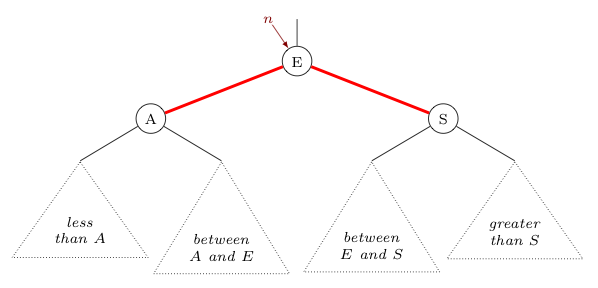private void flipColors(Node n) {

assert !isRed(n);        // only use this method when middle node n
assert isRed(n.left);    // has black parent connecting edge and both
assert isRed(n.right);   // edges connecting n to its children are red

n.color = RED;
n.left.color = BLACK;
n.right.color = BLACK;
}

Fixing "Left-Left Red" Edges"left-left red" edgesConsider the case where there are two adjacent red edges that span two levels, where the bottom and middle nodes are both left children of their parents, as shown at right.

This too is a 4-node that must be split, and have its middle key passed to the parent level.

Note that if we could perform an operation similar to left rotation, but in the other direction (appropriately called a right rotation) on the top node in this group, then we would have reduced things to a previous problem, where both of the adjacent red edges are on the same level. Following the right rotation with the previously described flip color operation will then complete the split and pass of the middle key to the parent level.

We first show the operation of right rotation below, and then we will show how this can be applied to a "left-left red" situation to deal with the corresponding 4-node.

Before Right RotationAfter Right Rotation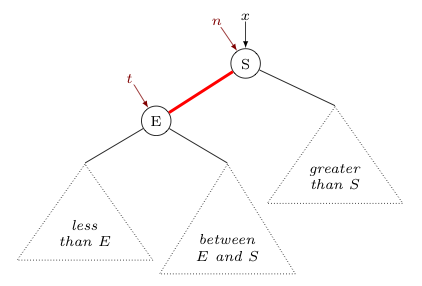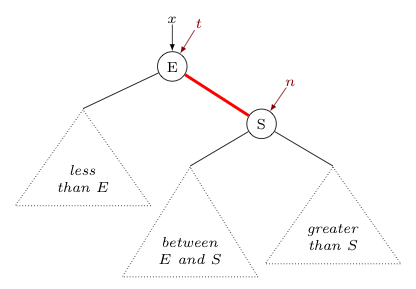private Node rotateRight (Node n) {

assert isRed(n.left)     // Use this method only if n's left child's edge is red.
// Note we don't check to see if n.left.left is red,
// although in the case described that is also true.
// The reason why is that this method will not only
// help us address the "left-left red" problem, but
// will also help us address a future problem as
// well -- one where n.left.left is not red.

Node t = n.left;
n.left = t.right;
t.right = n;
t.color = n.color;
n.color = RED;
return t;
}

...

// Similar to left rotation, suppose x is the parent reference to $S$.
// To update x, we invoke the following

x = rotateRight(x);

Now, let us see how right rotation and flipping colors can fix the "left-left red" problem, by first balancing and then splitting the underlying 4-node: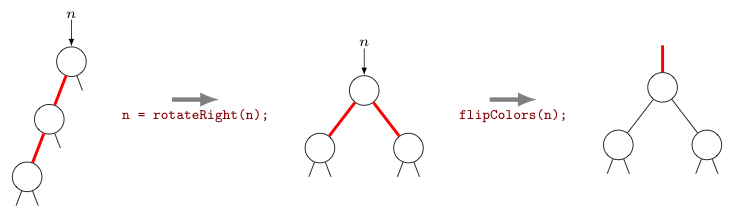Fixing "Left-Right Red" Edges"left-right red" edgesAs a final case to consider, suppose we again have two adjacent red edges that span two levels with the middle node a left child of its parent, but this time with the bottom node a right child of the middle node.

Just like the previous two cases, this again represents a 4-node in the associated 2-3 tree. So, our strategy is again to split the 4-node into two 2-nodes and pass the middle key to the former parent of the 4-node.

Fortunately, in this case, we don't need to develop a new operation to accomplish this. We can use the three actions described above (i.e., "left rotation", "right rotation", and "flip colors", in that order) to do this instead.

As can be seen below, the left rotation addresses the initially right-leaning red edge. Then, like the left-left red edge fix above, the right rotation balance the underlying 4-node, and the flip colors operation finishes the job -- splitting the 4-node and passing its middle key to its former parent.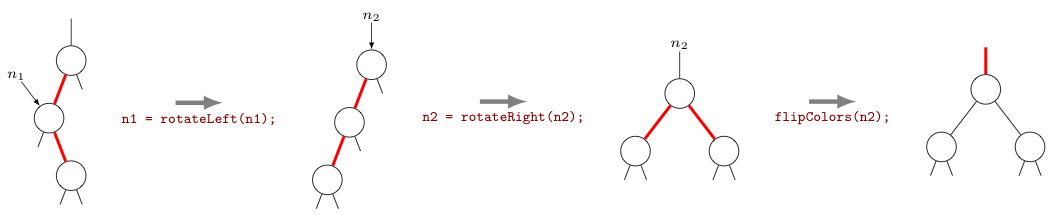The only tricky thing about the application of these operations in this context is that it first requires a reference to the middle node (i.e., $n_1$), and then a reference to the top node (i.e., $n_2$).

However, if one remembers how the recursive binary search tree method put() works, one will recall that we visit all of the nodes -- from the point of insertion all the way back to the root -- as references are "reset" as part of the recursive process.

Consequently, if we carefully inject our three operations during that upward-moving sequence of resets, we should be able to get access to those two nodes in the order we need them.

Putting Everything Together (and keeping the root black)

Often the operations described in the above section will eliminate a problem piece in a red-black tree in a single application. However, occasionally the red links that are created higher up in the tree as a result create problems of their own -- which must then be resolved as well.

Of course, this is completely consistent with how passing the middle key up to the parent of a temporary 4-node during the key insertion process in a 2-3 tree, can create additional 4-nodes higher in the tree that then also need to be eliminated.

In both cases, the strategy to resolve all of the problems is straight-forward. We simply continue resolving any problems seen, working upwards through the tree from the point of insertion towards the root.

If we should eliminate all of the problems before reaching the root, great! However, if problems persist all the way to the root, we'll need to consider one more thing.

Note that for three of the four problems that can occur (i.e., all but the lonely right-leaning red links), the solutions left us with a red edge above the top problem node.

Recalling again that a red edge directly above a node means the key in that node should be considered "glued to" the key in its parent node, combined with the fact that the root has no parent -- clearly there can never be a red edge above the root. Yet, if problems persist all the way to the root node, and if the last problem addressed is not a "lonely" right-leaning red link, that is exactly what will be produced.

The fix is simple in the extreme, though. As a last step in the insertion process, we just make sure the root is black. If we must change the color stored in the root node from red to black to ensure this, we can rest assured that no additional problems will be created -- as the last "flip colors" step taken will have made the two edges below the root node black as well.

With this in mind, we are now ready to present the following implementation of a put(Key key, Value val) method that can be used with red-black trees to insert new key-value pairs into the tree.

Of course, the code below assumes the instance methods rotateLeft(), rotateRight(), and flipColors() discussed above are all defined. The code shown in black is cannibalized from the binary search tree version of put(). Additional new code is shown in red.

As you examine this code, note in particular how tightly one is able to consolidate the application of operations used to resolve problem pieces (apart from the root). Amazingly, this can be implemented with only three if-statements! (Take a moment and apply the code to each of the previously discussed problems to convince yourself that these three statements accomplish everything done above.)

public void put(Key key, Value val) {
root = put(root, key, val);
root.color = BLACK;    // fix the root color, if other fixes made it red
}

private Node put(Node n, Key key, Value val) {
if (n = null) {
Node newNode = new Node(key, val, RED);
newNode.count = 1;
return newNode;
}

int cmp = key.compareTo(n.key);

if (cmp < 0)
n.left = put(n.left, key, val);
else if (cmp > 0)
n.right = put(n.right, key, val);
else
n.val = val;

if (isRed(n.right) && !isRed(n.left)) // fixes an initial right-leaning red edge n = rotateLeft(n); if (isRed(n.left) && isRed(n.left.left)) // balances a 4-node, as needed n = rotateRight(n); if (isRed(n.left) && isRed(n.right) // splits the 4-node, passing its middle flipColors(n); // key to its parent
n.count = 1 + size(n.left) + size(n.right); return n; }